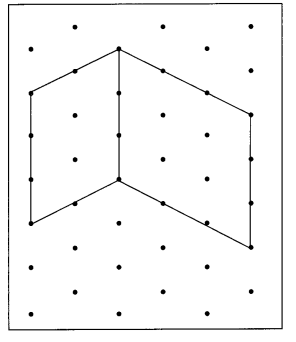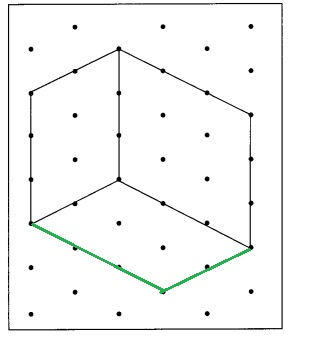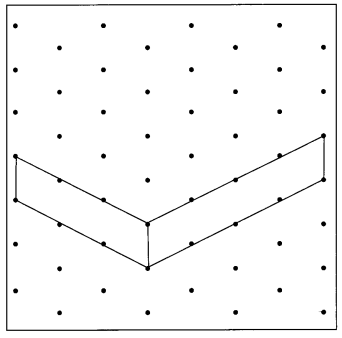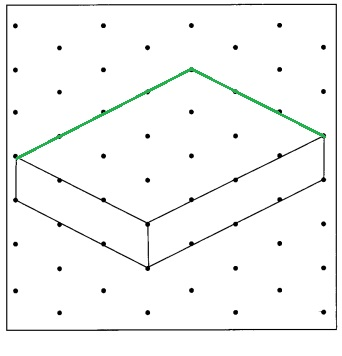# Math in Focus Grade 5 Chapter 15 Practice 2 Answer Key Drawing Cubes and Rectangular Prisms

This handy Math in Focus Grade 5 Workbook Answer Key Chapter 15 Practice 2 Drawing Cubes and Rectangular Prisms provides detailed solutions for the textbook questions.

## Math in Focus Grade 5 Chapter 15 Practice 2 Answer Key Drawing Cubes and Rectangular Prisms

Draw on dot paper.

Question 1.
Draw a unit cube.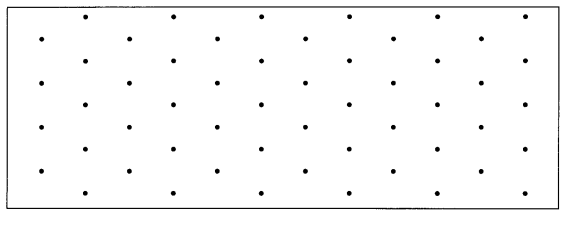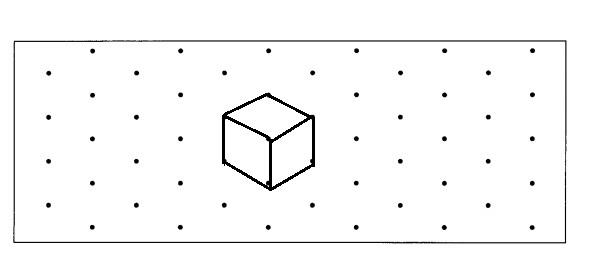Explanation:
As a cube has all its sides of the same length.
A unit cube has all its sides of length 1 unit.
Shown the volume of a unit cube = Side × Side × Side,
= 1 unit × 1 unit × 1 unit,
= 1 unit cubes.

Question 2.
Draw two different views of a rectangular prism made up of 2 unit cubes.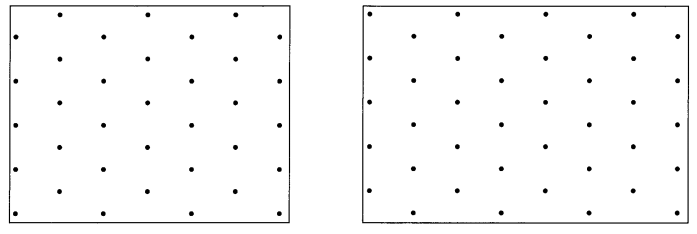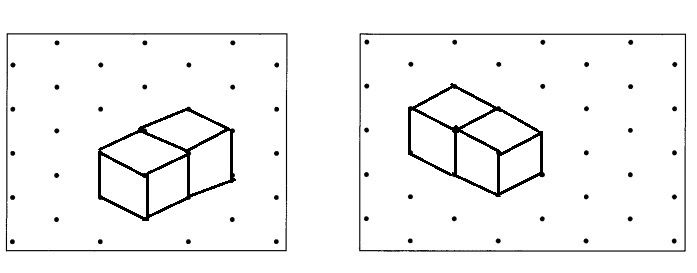Explanation:
Drawn two different views of a rectangular prism made up of 2 unit cubes,
A unit cube has all its sides of length 1 unit
and 2 unit cubes shows the volume of a 2 unit cubes = 2 X (Sides × Side × Side),
= 2 X (1 unit × 1 unit × 1 unit),
= 2 unit cubes.

Question 3.
Draw two different solids made up of 3 unit cubes each.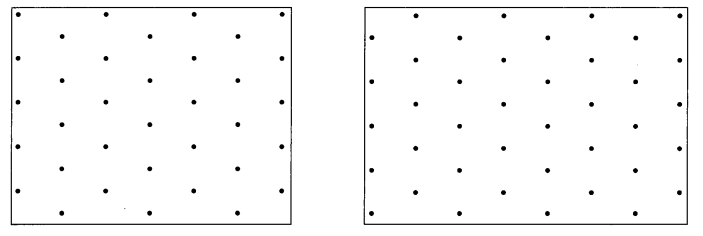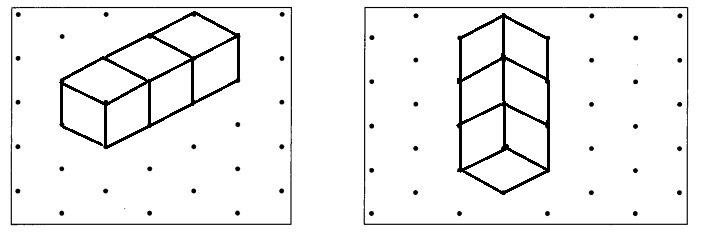Explanation:
Drawn two different views of a rectangular prism made up of 3 unit cubes,
A unit cube has all its sides of length 1 unit
and 3 unit cubes shows the volume of a 3 unit cubes = 3 X (Sides × Side × Side),
= 3 X (1 unit × 1 unit × 1 unit),
= 3 unit cubes.

Draw each cube or rectangular prism on the dot paper.

Example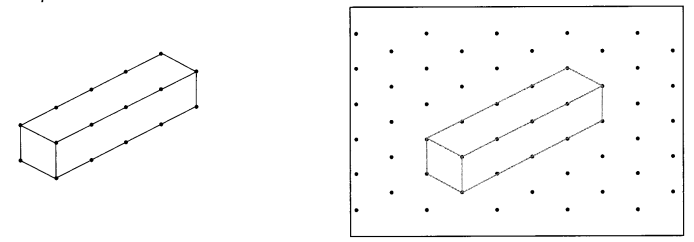Question 4.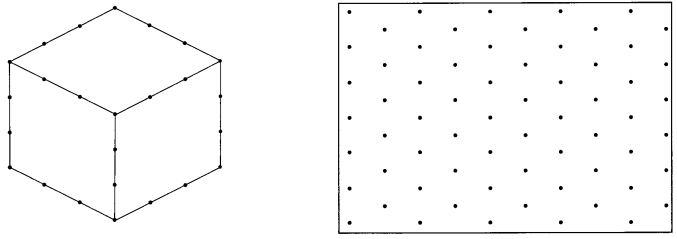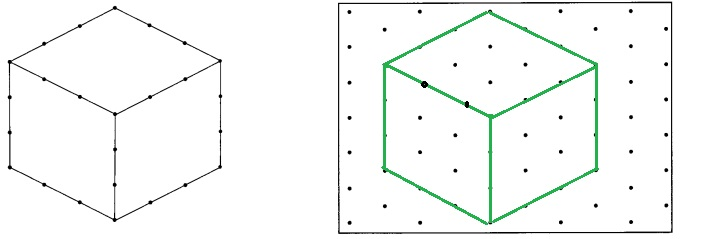Explanation:
Drawn one cube on the dot paper,
As given cube has 4 units shown the volume of cube =
side X side X side = 4 units X 4 units X 4 units = 64 unit cubes.

Question 5.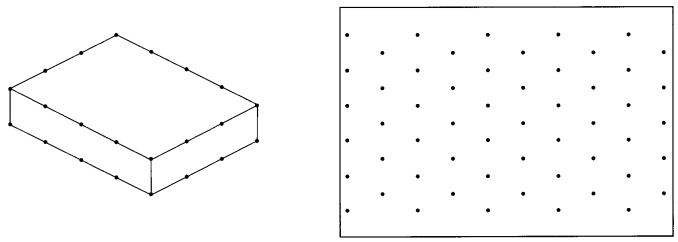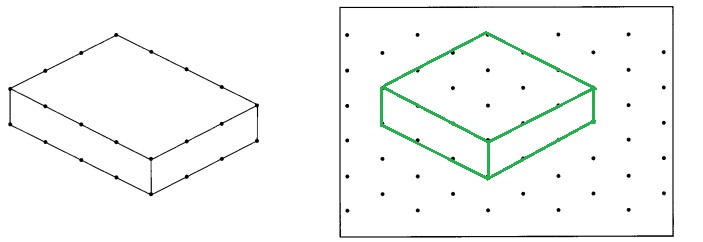Explanation:
Drawn one rectangular prism on the dot paper,
As given rectangular prism has 4 units X 4 units  X 1 unit =
16 unit cubes rectangular prism.

Question 6.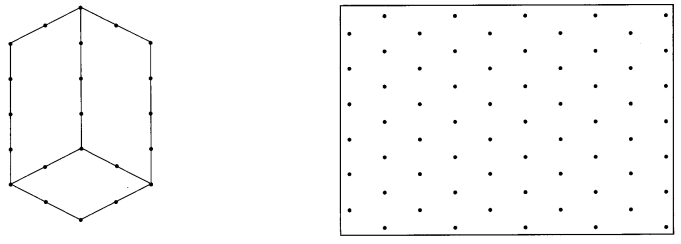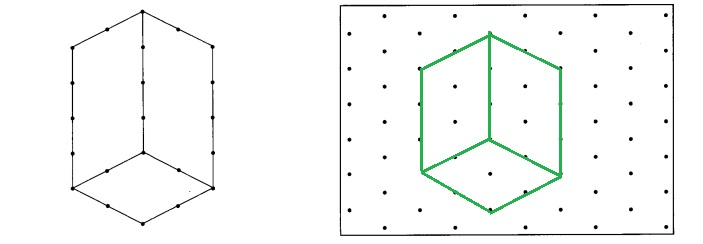Explanation:
Drawn one rectangular prism on the dot paper,
As given rectangular prism has 4 units X 2 units  X 2 units =
16 unit cubes rectangular prism.

Draw each cube or rectangular prism on the dot paper.

Question 7.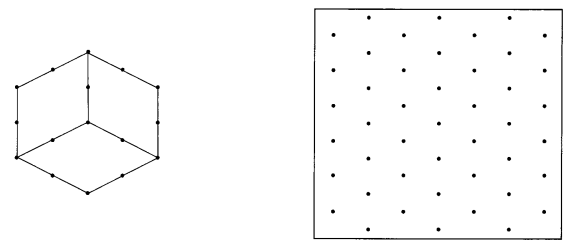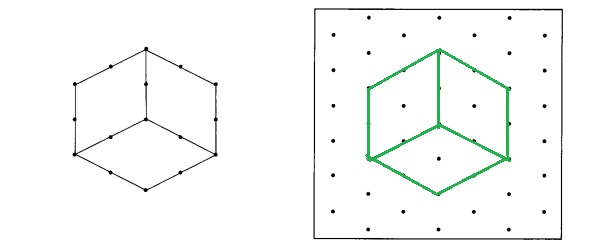Explanation:
Drawn one rectangular prism on the dot paper,
As given rectangular prism has 2 units X 2 units  X 2 units =
8 unit cubes rectangular prism.

Question 8.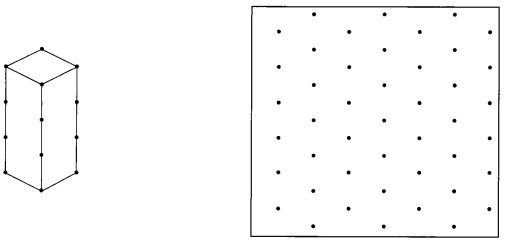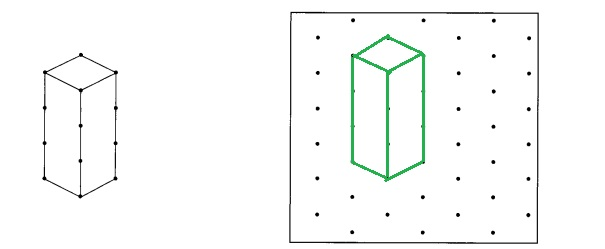Explanation:
Drawn one rectangular prism on the dot paper,
As given rectangular prism has 3 units X 1 unit  X 1 unit =
3 unit cubes rectangular prism.

Draw a cube with edges 4 times as long as the edges of this unit cube.

Question 9.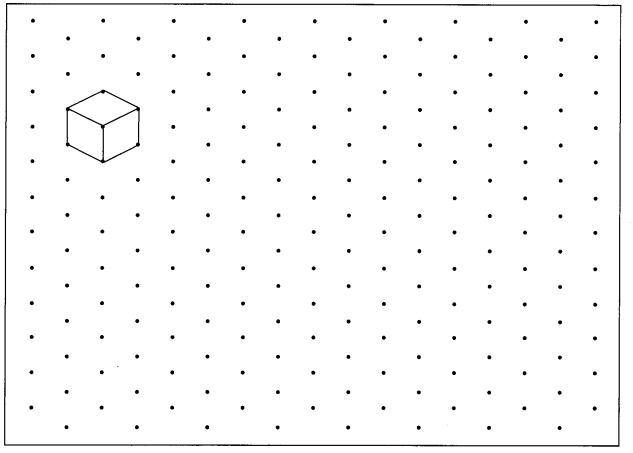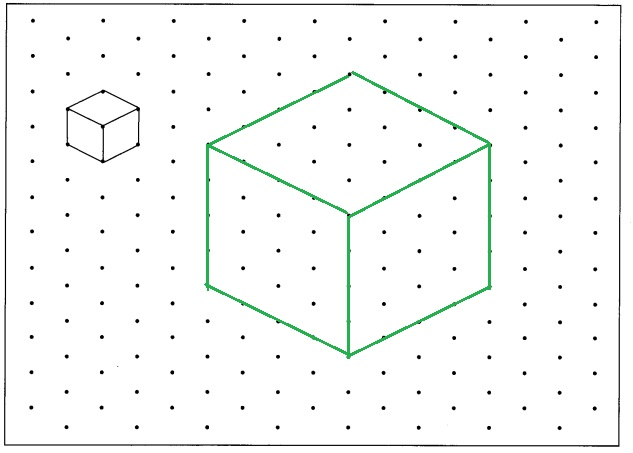Explanation:
Given 1 unit cube with volume 1 unit X 1 unit X 1 unit = 1 cubic unit and
Drawn a cube with edges 4 times as long as the given edge so the volume is
4 units X 4 units X 4 units = 64 cubic units.

Complete the drawing of each cube or rectangular prism.

Question 10.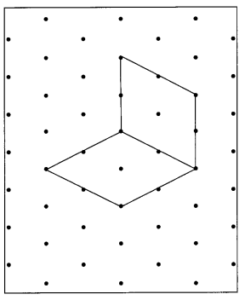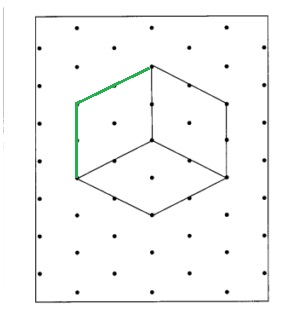Explanation:
Completed the drawing of given cube as shown above
which has 2 units.

Question 11.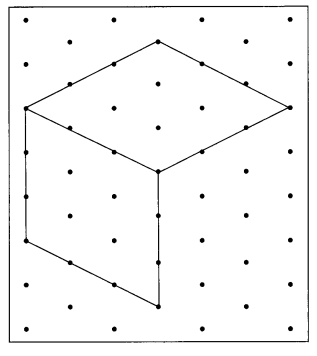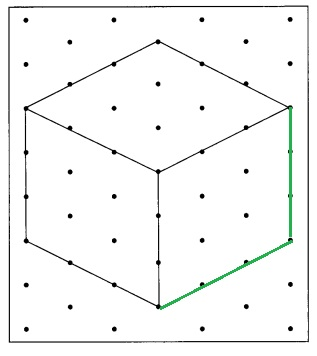Explanation:
Completed the drawing of given cube as shown above
which has 3 units.

Question 12.